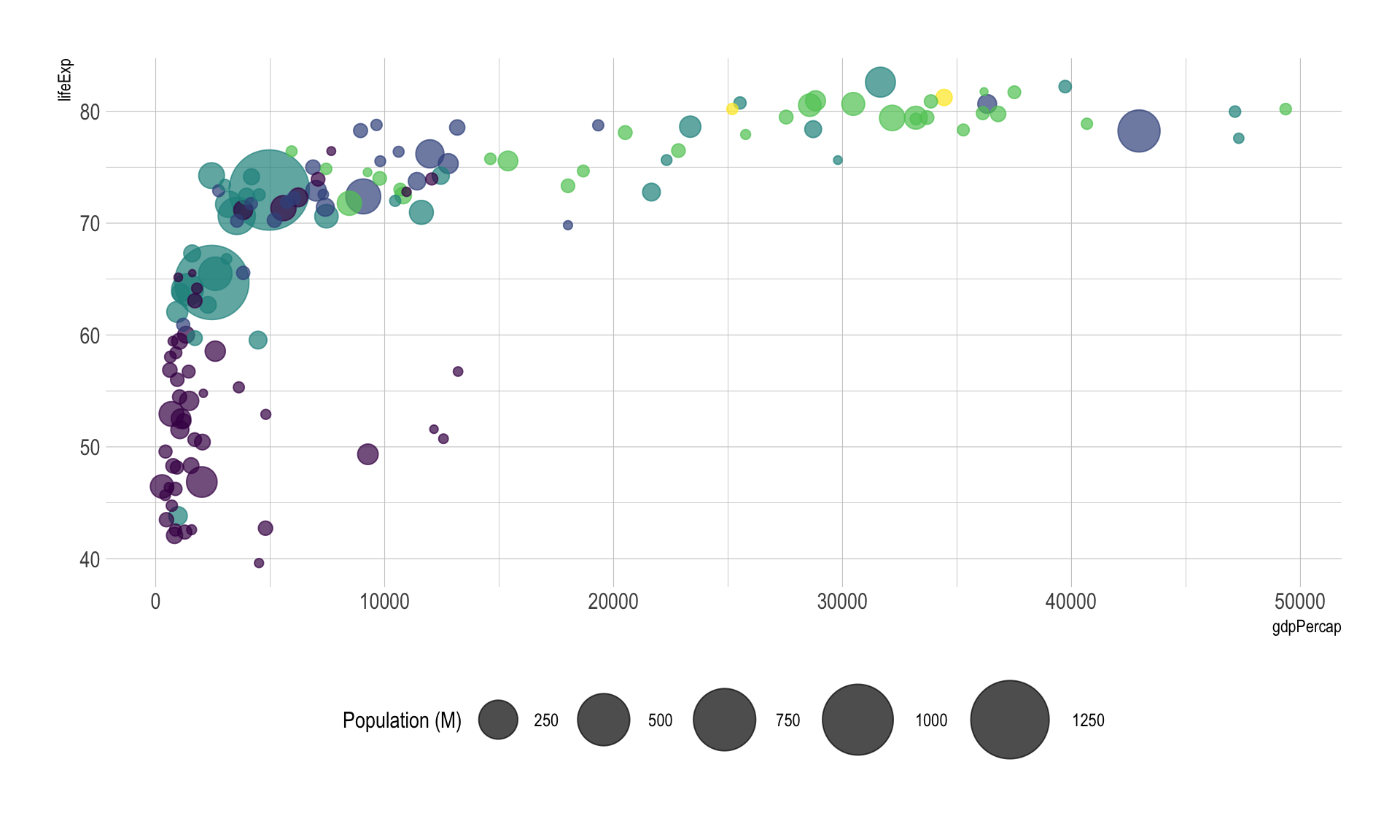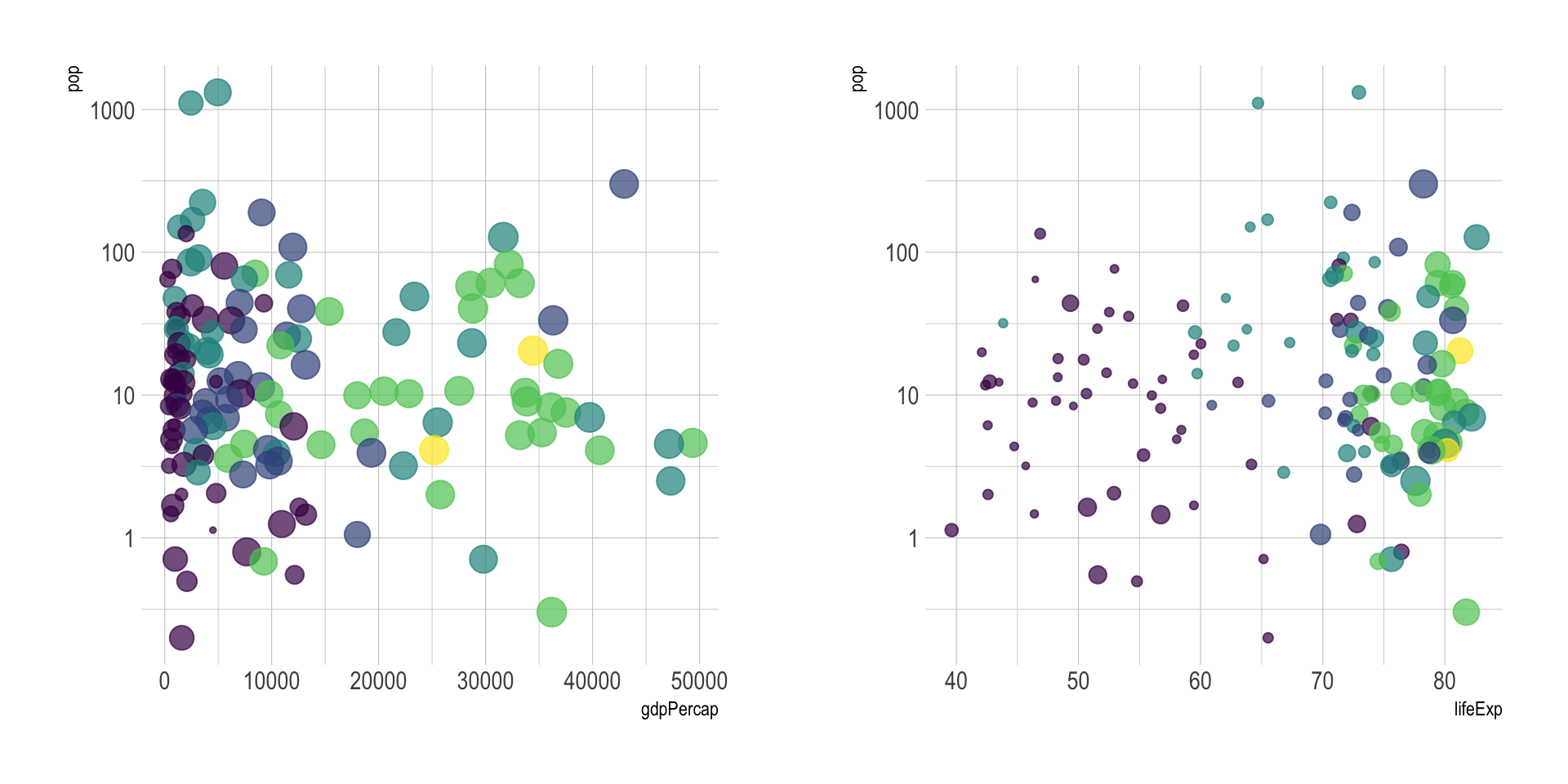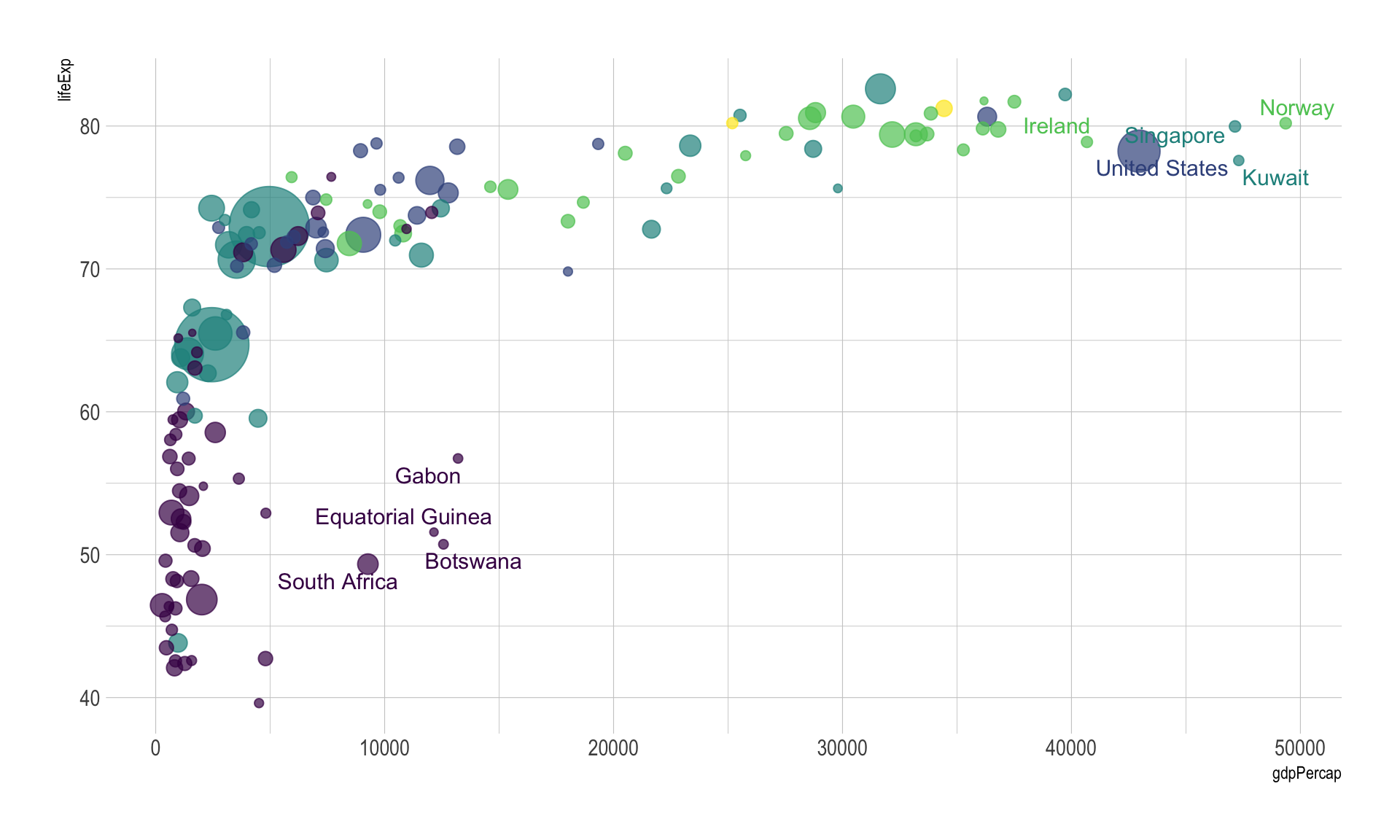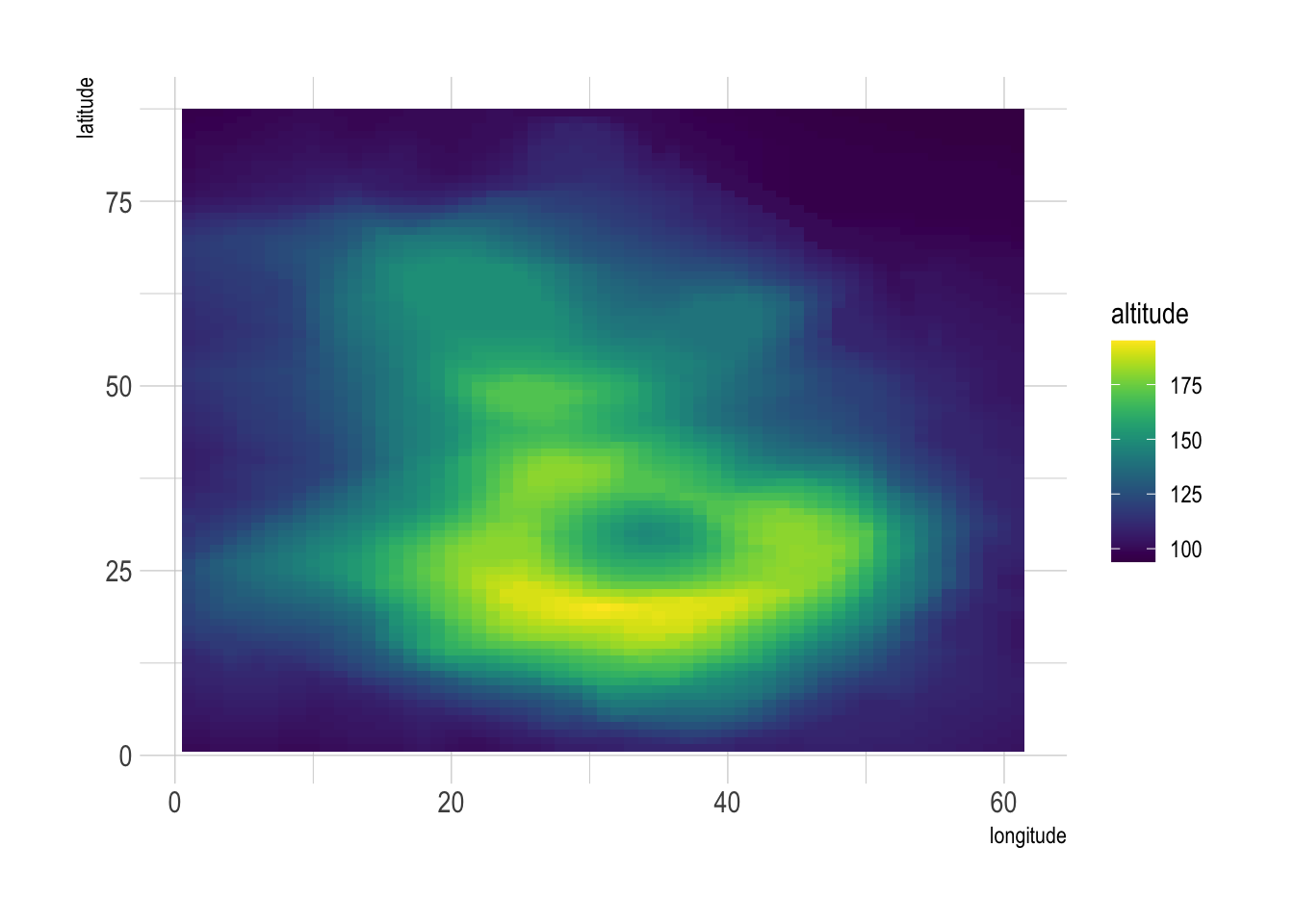This document gives a few suggestions to analyse a dataset composed by `three numeric variables`. It considers an abstract of the Gapminder dataset made famous through the Hans Rosling Ted Talk. It provides the average life expectancy, gdp per capita and population size for more than 100 countries. This dataset is available through the `gapminder` R package and you can download it here. The table beside shows a glimpse of it.

``````# Libraries
library(tidyverse)
library(hrbrthemes)
library(viridis)
library(kableExtra)
options(knitr.table.format = "html")
library(plotly)
library(gridExtra)
library(ggrepel)

# The dataset is provided in the gapminder library
library(gapminder)
data <- gapminder %>% filter(year=="2007") %>% select(-year)

# show data
mutate(gdpPercap=round(gdpPercap,0)) %>%
mutate(pop=round(pop/1000000,2)) %>%
mutate(lifeExp=round(lifeExp,1)) %>%
kable() %>%
kable_styling(bootstrap_options = "striped", full_width = F)``````
country continent lifeExp pop gdpPercap
Afghanistan Asia 43.8 31.89 975
Albania Europe 76.4 3.60 5937
Algeria Africa 72.3 33.33 6223
Angola Africa 42.7 12.42 4797
Argentina Americas 75.3 40.30 12779
Australia Oceania 81.2 20.43 34435

# Bubble plot

The go to graph in that kind of situation is probably the bubble plot. The bubble plot is really close to a scatterplot since it shows the value of two numeric variables on the X and Y axis. However, it allows to study the value of a third variable using different sizes for the dots (that are thus called bubbles).

``````# Show a bubbleplot
data %>%
mutate(pop=pop/1000000) %>%
arrange(desc(pop)) %>%
mutate(country = factor(country, country)) %>%
ggplot( aes(x=gdpPercap, y=lifeExp, size = pop, color = continent)) +
geom_point(alpha=0.7) +
scale_size(range = c(1.4, 19), name="Population (M)") +
scale_color_viridis(discrete=TRUE, guide=FALSE) +
theme_ipsum() +
theme(legend.position="bottom")``````The problem with bubble plot is that the relationship between the variable of the X and Y axis is much more obvious than the relationship with the third variable. Thus you must prioritize your variables and be sure of what you want to show. Before doing that kind of chart, I believe it is a good practice to try other combinations:

``````p2 <- data %>%
mutate(pop=pop/1000000) %>%
arrange(desc(pop)) %>%
mutate(country = factor(country, country)) %>%
ggplot( aes(x=gdpPercap, y=pop, size = lifeExp, color = continent)) +
geom_point(alpha=0.7) +
scale_color_viridis(discrete=TRUE) +
scale_y_log10() +
theme_ipsum() +
theme(legend.position="none")

p3 <- data %>%
mutate(pop=pop/1000000) %>%
arrange(desc(pop)) %>%
mutate(country = factor(country, country)) %>%
ggplot( aes(x=lifeExp, y=pop, size = gdpPercap, color = continent)) +
geom_point(alpha=0.7) +
scale_color_viridis(discrete=TRUE) +
scale_y_log10() +
theme_ipsum() +
theme(legend.position="none")

grid.arrange(p2,p3, ncol=2)``````In this case there is no obvious relationship between opulation and other metrics so it makes sense to use population for the bubble size.

If you just want to highlight the relationship between gbp per capita and life Expectancy you’ve probably done most of the work now. However, it is a good practice to highlight a few interesting dots in this chart to give more insight to the plot:

``````# Prepare data
tmp <- data %>%
mutate(
annotation = case_when(
gdpPercap > 5000 & lifeExp < 60 ~ "yes",
lifeExp < 30 ~ "yes",
gdpPercap > 40000 ~ "yes"
)
) %>%
mutate(pop=pop/1000000) %>%
arrange(desc(pop)) %>%
mutate(country = factor(country, country))

# Plot
ggplot( tmp, aes(x=gdpPercap, y=lifeExp, size = pop, color = continent)) +
geom_point(alpha=0.7) +
scale_size(range = c(1.4, 19), name="Population (M)") +
scale_color_viridis(discrete=TRUE) +
theme_ipsum() +
theme(legend.position="none") +
geom_text_repel(data=tmp %>% filter(annotation=="yes"), aes(label=country), size=4 )``````Last but not least, note that bubble plot is probably the type of chart where using interactivity makes the more sense. In the following plot you can hover bubbles to get more information and zoom on a specific part of the graphic.

``````# Interactive version
p <- data %>%
mutate(gdpPercap=round(gdpPercap,0)) %>%
mutate(pop=round(pop/1000000,2)) %>%
mutate(lifeExp=round(lifeExp,1)) %>%
arrange(desc(pop)) %>%
mutate(country = factor(country, country)) %>%
mutate(text = paste("Country: ", country, "\nPopulation (M): ", pop, "\nLife Expectancy: ", lifeExp, "\nGdp per capita: ", gdpPercap, sep="")) %>%
ggplot( aes(x=gdpPercap, y=lifeExp, size = pop, color = continent, text=text)) +
geom_point(alpha=0.7) +
scale_size(range = c(1.4, 19), name="Population (M)") +
scale_color_viridis(discrete=TRUE, guide=FALSE) +
theme_ipsum() +
theme(legend.position="none")

ggplotly(p, tooltip="text")``````

# A note on the grid scenario

A specific use case where three numeric columns are displayed is the grid system. In this case, the two first columns gives the grid coordinates, and the third variable gives a numeric value for each position of the grid. For example, the volcano data set provides that altitude of each point of the grid of a volcano:

``````# prepare the dataset:
don <- volcano
colnames(don) <- seq(1,ncol(don))
don <- don %>%
as.tibble() %>%
mutate(lat=seq(1,nrow(don)) ) %>%
gather(key="long", value="altitude", -lat)

# show data
don %>% head(6) %>% kable() %>%
kable_styling(bootstrap_options = "striped", full_width = F)``````
lat long altitude
1 1 100
2 1 101
3 1 102
4 1 103
5 1 104
6 1 105

This kind of data can be represented using a heatmap (2d):

``````don %>%
na.omit() %>%
ggplot(aes(x=as.numeric(long), y=lat, fill=altitude)) +
geom_tile() +
scale_fill_viridis() +
theme_ipsum() +
xlab("longitude") +
ylab("latitude")``````Another way is to build a surface plot. It really makes sense to use 3D in this special case since it allows to visualize the real shape of the volcano:

``plot_ly(z = volcano, type = "surface")``

# Going further

You can learn more about each type of graphic presented in this story in the dedicated sections. Click the icon below: